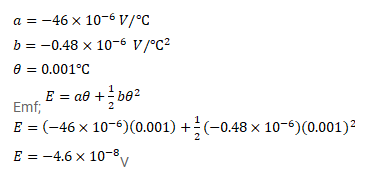# The temperatures of the junctions of a bismuth-silver

Question:

The temperatures of the junctions of a bismuth-silver thermocouple are maintained at $0^{\circ} \mathrm{C}$ and $0.0011^{\circ} \mathrm{C}$. Find the thermo-emf (Seebeck emf) developed. For Bismuth-silver, $a=-46 \times 10^{-6} \mathrm{~V} / \mathrm{deg}$ and $b=-0.48 \times 10^{-6} \mathrm{~V} / \mathrm{deg}^{2}$.

Solution: